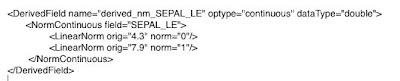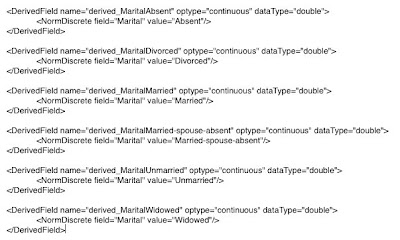# How are data transformations represented in PMML?April 22, 2009
934 Views

PMML supports several kinds of data transformations. Below, we list the most common together with examples.

Data transformations involved in the pre-processing of the input variables/fields are mainly located inside the following PMML elements: TransformationDictionary and LocalTransformations.

For the formal PMML schema definition of the transformations covered here, please refer to the PMML Transformations page on the DMG website.

Value Mapping

Value mapping can be used to map discrete values to discrete values. The example below shows how to map a categorical field (color) into a numerical field (derived_color).Note that in this example, we are mapping yellow to 3, white to 1, blue to 6, and green to 4.

The original input field named color needs to be defined in the DataDictionary element. The derived field, the result of our data transformation is now called derived_color. This field can subsequently be used by the model as an input variable or used as input for another data transformation.

Discretization

Discretization is used to map continuous values to discrete values. The example below shows how to discretize a continuous field (units) into a discrete field (derived_units).

PMML supports several kinds of data transformations. Below, we list the most common together with examples.

Data transformations involved in the pre-processing of the input variables/fields are mainly located inside the following PMML elements: TransformationDictionary and LocalTransformations.

For the formal PMML schema definition of the transformations covered here, please refer to the PMML Transformations page on the DMG website.

Value Mapping

Value mapping can be used to map discrete values to discrete values. The example below shows how to map a categorical field (color) into a numerical field (derived_color).Note that in this example, we are mapping yellow to 3, white to 1, blue to 6, and green to 4.

The original input field named color needs to be defined in the DataDictionary element. The derived field, the result of our data transformation is now called derived_color. This field can subsequently be used by the model as an input variable or used as input for another data transformation.

Discretization

Discretization is used to map continuous values to discrete values. The example below shows how to discretize a continuous field (units) into a discrete field (derived_units).In this example, we are transforming an interval to a discrete value, more specifically, discretize will transform [1,2[ to 1, [2,3[ to 2, and [3,100] to 3.

The new field is now called derived_units and can be used as input to another transformation or to the model itself.

Normalization

As specified in the DMG website, normalization provides a basic framework for mapping input values to specific value ranges, usually the numeric range [0 .. 1].

NormContinuous

Normalization is used, e.g., in neural networks. In fact, if you export your neural network model using SPSS (starting with version 16), the PMML code generated will contain this kind of transformation for the neural inputs. The R PMML package will also generate a file containing the normalization of input variables for Support Vector Machines (SVMs). The example below was extracted from the Iris_SVM.xml file available in the Zementis website.The PMML element NormContinuous can be used to implement simple normalization functions such as the z-score transformation (X – m ) / s, where m is the mean value and s is the standard deviation.

NormDiscrete

The NormDiscrete element is used to implement the dummyfication of categorical or ordinal fields. For example, if you have a categorical variable called Marital with the following possible values: Absent, Divorced, Married, Married-spouse-absent, Unmarried, and Widowed, you may want these to be dummyfied (i.e. translated into 0s and 1s) for use by a neural network or SVM. The example below shows the use of element NormDiscrete to accomplish just that.The set of NormDiscrete instances which refer to input field Marital define a fan-out function which maps a single input field to a set of normalized fields. Note that if Marital is equal to Married, the field derived_MaritalMarried will be assigned a value equals to 1.0 and all other derived_MaritalX fields shown will be assigned values equal to 0.

This code was extrated from the Audit_SVM.xml file available in the Zementis website. It is automatically exported by the R PMML package for SVMs built using the R ksvm (kernlab) package.

Functions

PMML offers several built-in functions, all of which are supported by ADAPA. The list is as follows:

1. +, -, * and /
2. min, max, sum and avg
3. log10, ln, sqrt, abs, exp, pow, threshold, floor, ceil, round
4. uppercase
5. substring
6. trimBlanks
7. formatNumber
8. formatDatetime
9. dateDaysSinceYear
10. dateSecondsSinceYear
11. dateSecondsSinceMidnight

You can find several examples of the use of such functions in the DMG website.

Note that functions such as min, max, sum and avg take a variable number of parameters (derived fields or input fields) and return a single value which you would then assign to a new derived field.

Comprehensive blog featuring topics related to predictive analytics with an emphasis on open standards, Predictive Model Markup Language (PMML), cloud computing, as well as the deployment and integration of predictive models in any business process.